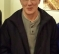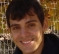## Does group delay only match real filter delay ONLY inside the passband?Started by 6 years ago3 replieslatest reply 6 years ago131 views

When filtering a sinusoid with an IIR filter (50 Hz cutoff- highpass filter, Fs=48K ) I observed that the group delay that matlab shows me does not match the actual delay at the output. This is true when the sinusoid is close or below the cutoff frequency, like 20-80 Hz. I wonder what is the reason?

[ - ]For IIR filters, group delay is notoriously difficult to measure at frequency response transition regions.  Phase unwrapping has to be performed carefully and I don't know if there's a perfect algorithm for it, I think it's always done a bit ad-hoc.

I'm not sure what MATLAB is showing you, but here is an elliptic bandpass filter response showing unwrapped phase and group delay, calculated using an FFT applied to the filter impulse response:as you can see getting an accurate value in transition regions might be challenging.  A lot would depend on how you're measuring "actual delay" -- size of FFT to calculate frequency response, algorithm for phase unwrapping, numerical accuracy, etc.

-Jeff

[ - ]Forgive me if I am saying things you already known. Group delay is not constant for IIR filter. It is a function of the frequency. Therefore, your filter will produce a different group delay for each frequency component of the input signal. Group delay is the derivative of your filter phase vs frequency response, which can be calculated analytically for a specific frequency value.

The phase response is given by

$$\phi(\omega) = \arg\left \{ H(i\omega) \right \}$$

where $$H(i\omega)$$ is the filter transfer function.

The group delay is given by

$$\tau(\omega) = \frac{d \phi(\omega)}{d \omega}$$

Regards

[ - ]